C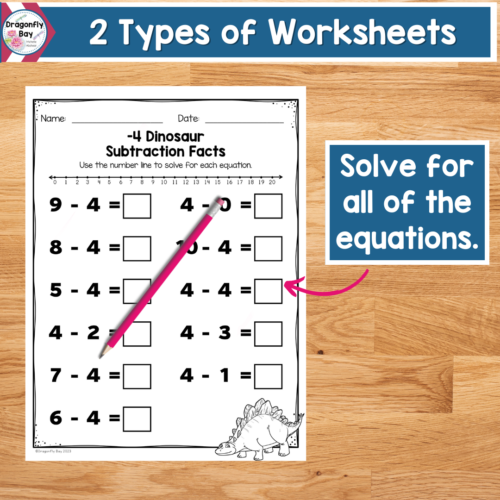### Details

Languages
English
Kindergarten, 1st, 2nd
Resource Type
Printables, Worksheets
Product
Digital
Pages
70 Pages
Subject
Subtraction
Standard
Common Core Mathematics Standards

### Description

Do your students need to practice their mental math skills? Are they needing to improve their accuracy and speed in Math? Practice subtraction fluency facts with this dinosaur themed 70 page printable set. Students will focus on subtracting 0-10 math facts using a number line.

There are 2 differentiated types of worksheets included.
The first worksheet type has students model each equation on a number line.
The second worksheet type has students use a number line to solve for all of the equations. Each of the focus subtraction facts has a different dinosaur themed page.

This is great for Kindergarten, First Grade or as a review for Second Grade. This will also help students that need more practice at home or with homeschooling.

✨MY PRODUCT INCLUDES✨
70 page pdf
2 types of worksheets (model on the number line, use a number line to solve)
All subtraction equations from 0-10 with each page focusing on a specific number (-5).

THIS PRODUCT FOCUSES ON THE FOLLOWING STANDARDS:

✅Common Core State Standards
K.OA.A.5 Fluently add and subtract within 5.
1.OA.C.6 Add and subtract within 20, demonstrating fluency for addition and subtraction within 10. Use strategies such as counting on; making ten (e.g., 8 + 6 = 8 + 2 + 4 = 10 + 4 = 14); decomposing a number leading to a ten (e.g., 13 – 4 = 13 – 3 – 1 = 10 – 1 = 9); using the relationship between addition and subtraction (e.g., knowing that 8 + 4 = 12, one knows 12 – 8 = 4); and creating equivalent but easier or known sums (e.g., adding 6 + 7 by creating the known equivalent 6 + 6 + 1 = 12 + 1 = 13).
2.OA.B.2 Fluently add and subtract within 20 using mental strategies. By end of Grade 2, know from memory all sums of two one-digit numbers.

✅Texas Essential Knowledge and Skills
MA.K.3.A Number and operations. The student applies mathematical process standards to develop an understanding of addition and subtraction situations in order to solve problems. The student is expected to: model the action of joining to represent addition and the action of separating to represent subtraction;
MA.1.3.D Number and operations. The student applies mathematical process standards to develop and use strategies for whole number addition and subtraction computations in order to solve problems. The student is expected to: apply basic fact strategies to add and subtract within 20, including making 10 and decomposing a number leading to a 10;
MA.2.4.A Number and operations. The student applies mathematical process standards to develop and use strategies and methods for whole number computations in order to solve addition and subtraction problems with efficiency and accuracy. The student is expected to: recall basic facts to add and subtract within 20 with automaticity;

✅Virginia Standards of Learning
CE.1.7.b The student will demonstrate fluency with addition and subtraction within 10.
CE.2.5.b The student will demonstrate fluency with addition and subtraction within 20.

QUESTIONS OR CONNECT WITH ME

✉ EMAIL

[email protected]

✍MESSAGE ME:

www.dragonfly-bay.com

www.dragonflybaylearning.com

❤️Thank you,

Michelle

Dragonfly Bay

Do your students need to practice their mental math skills? Are they needing to improve their accuracy and speed in Math? Practice subtraction fluency facts with this dinosaur themed 70 page printable set. Students will focus on subtracting 0-10 math... more

### Reviews

0
Overall review score

Displaying All Reviews | 0 Reviews

0
0%
0
0%
0
0%
0
0%
0
0%

### More from this shop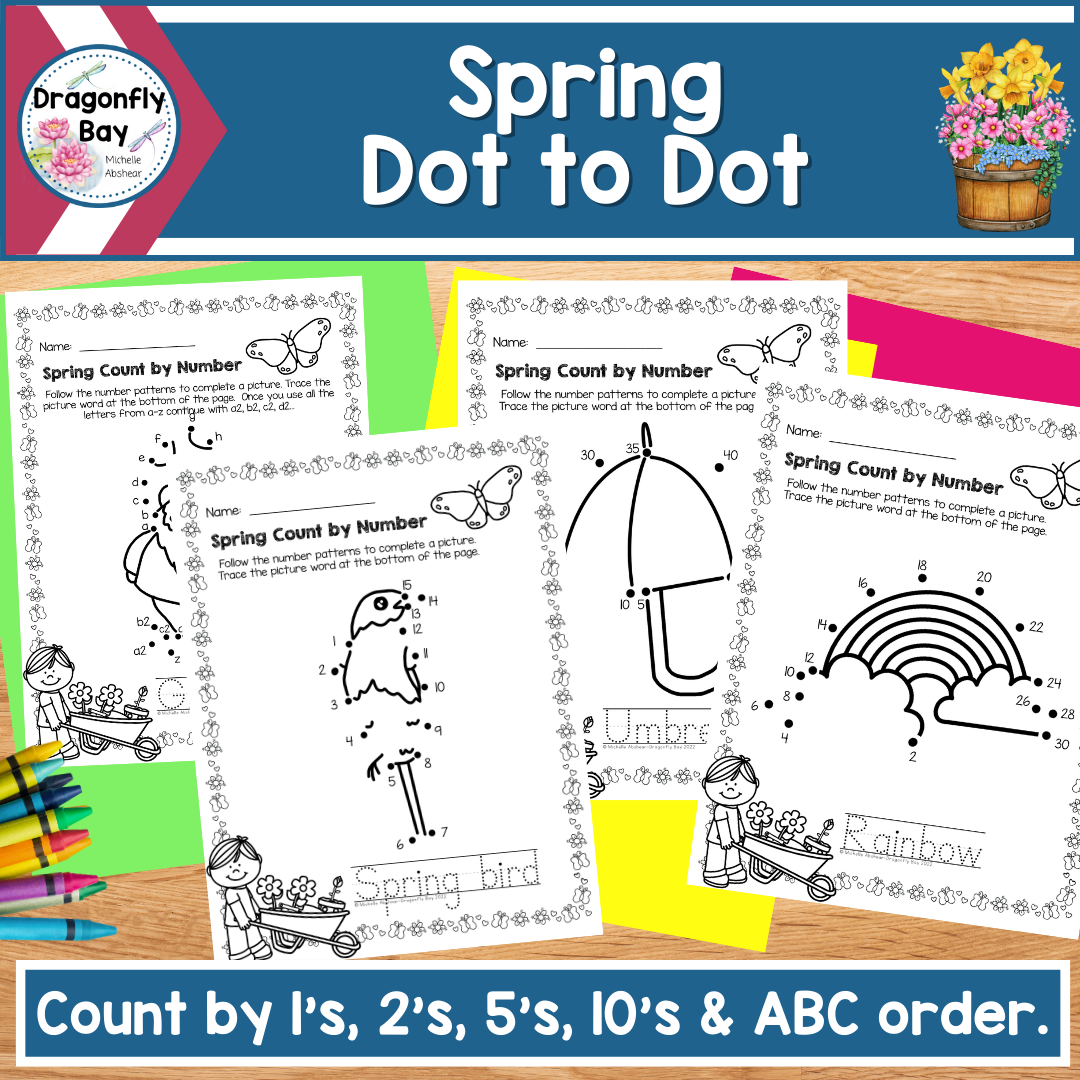\$6.00\$4.00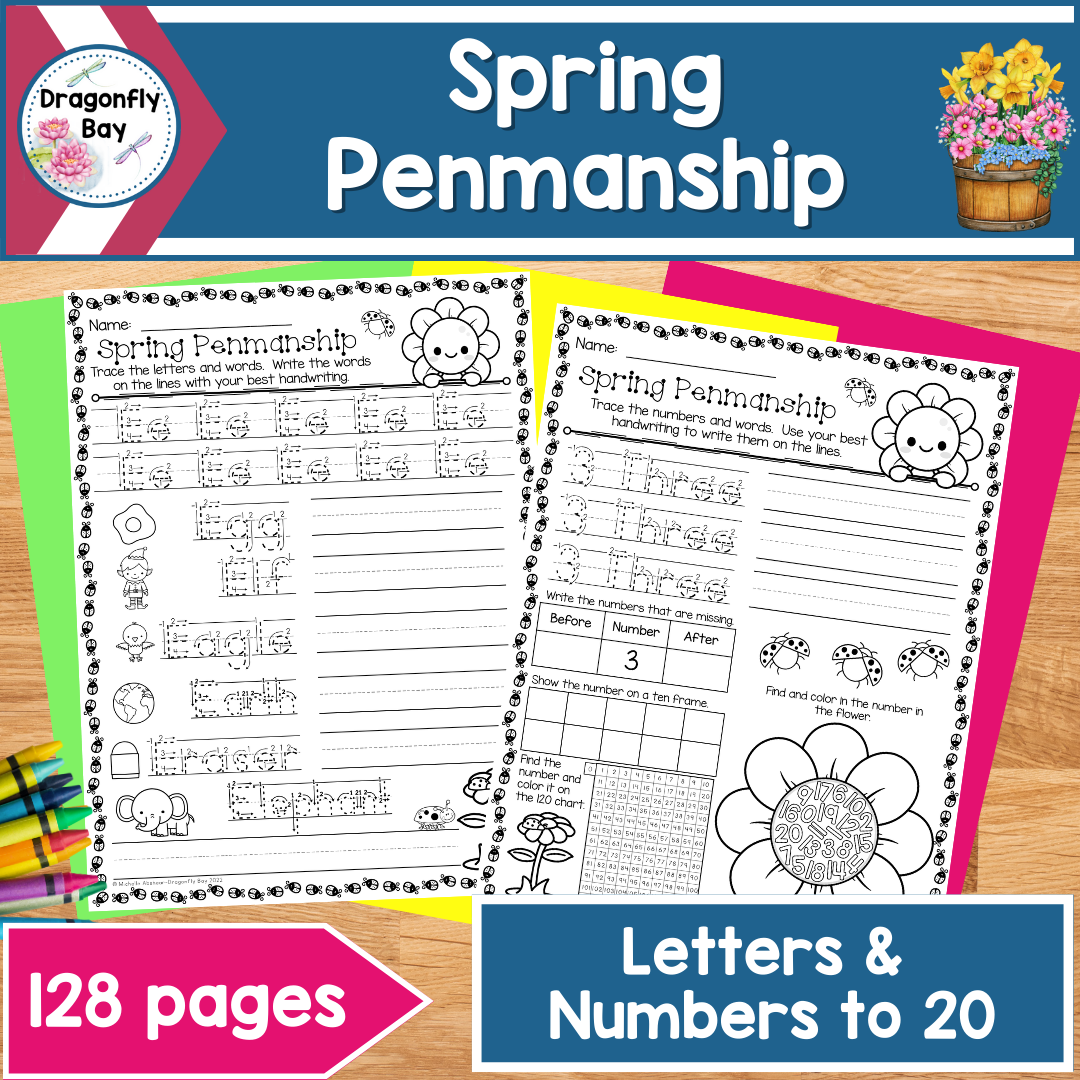\$6.00Free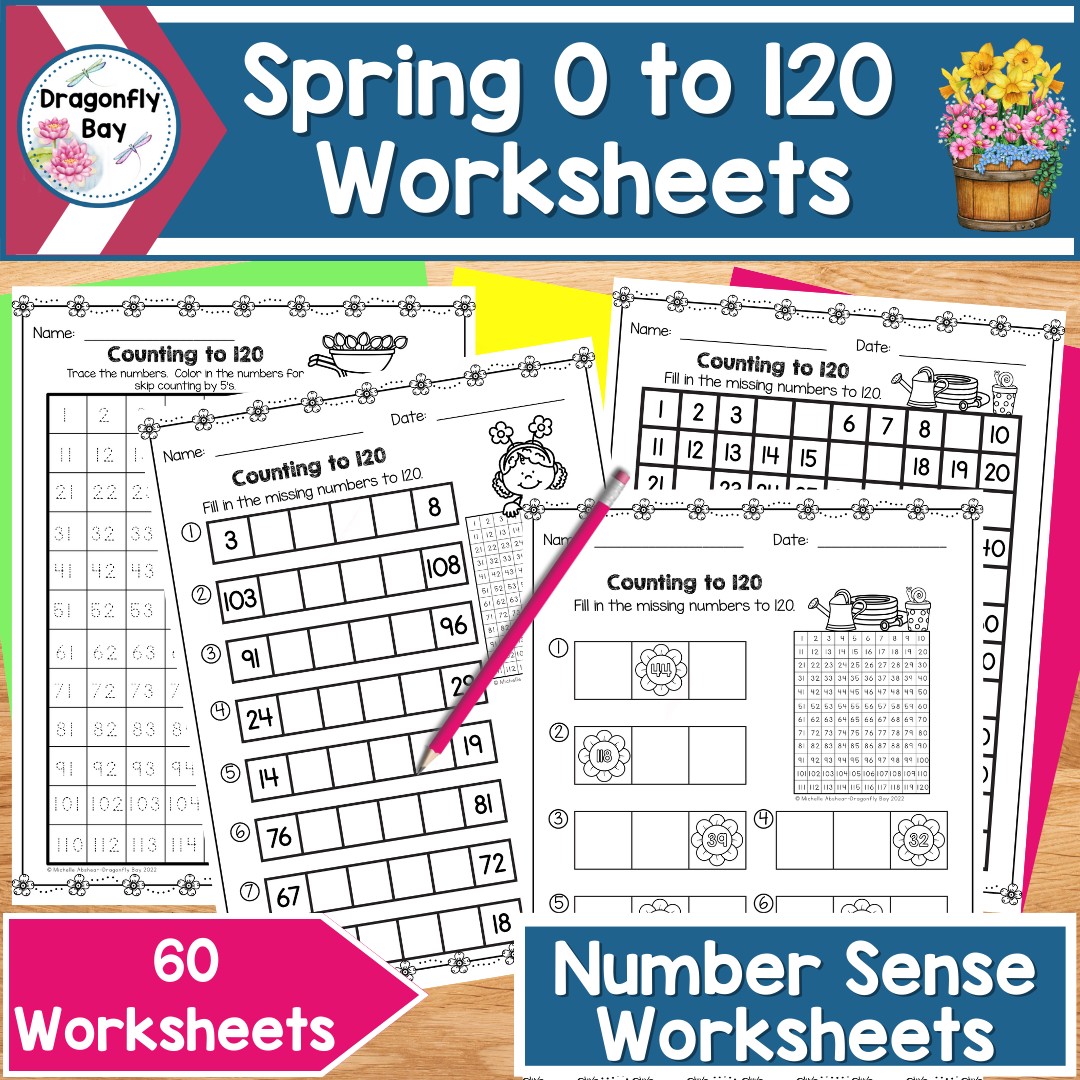\$5.00\$6.00\$4.00\$2.00\$4.50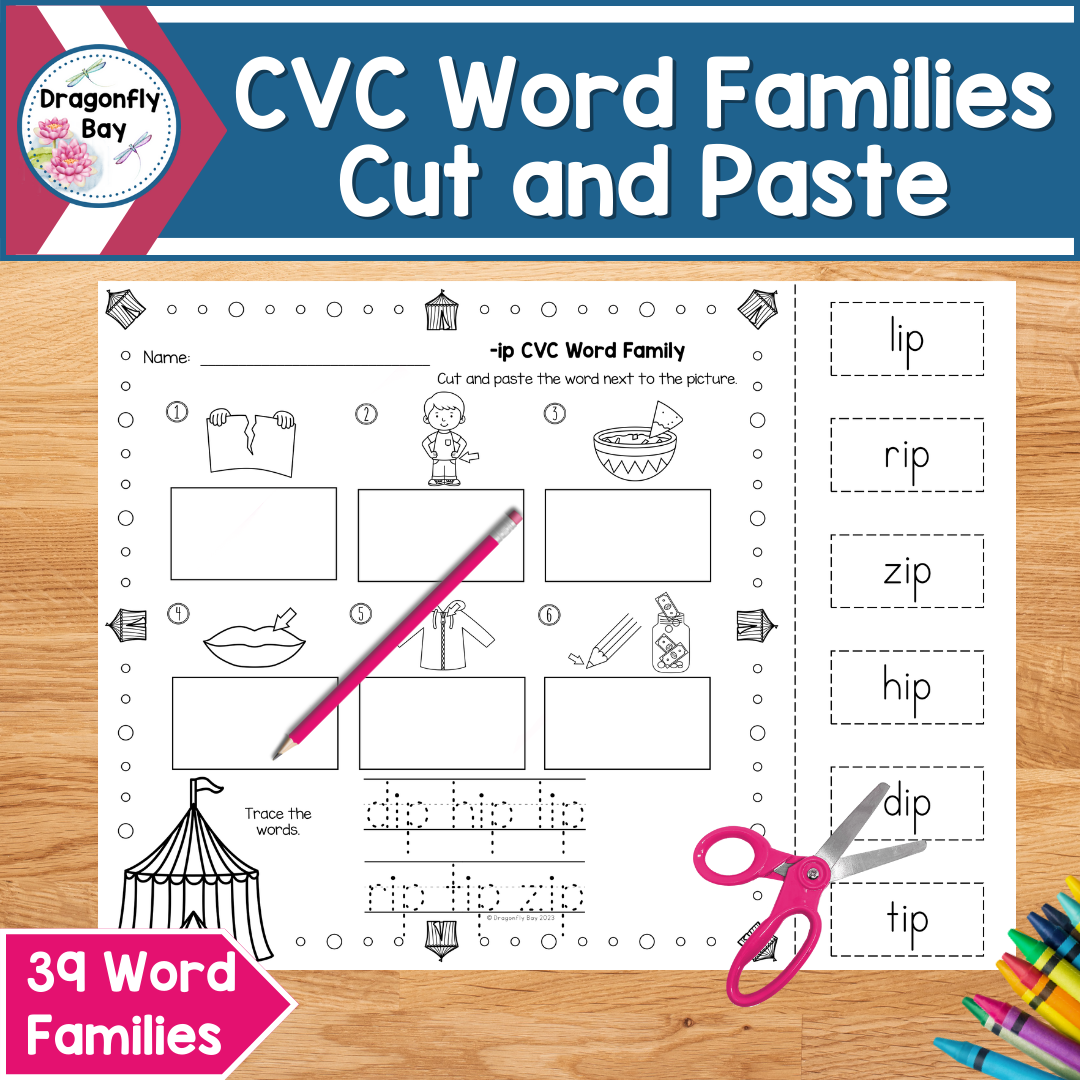\$3.00\$2.00\$4.00\$4.00\$6.00\$6.00

Shop | Product

# Subtraction Fluency 0-10 Facts with a Number Line Dinosaur Themed

Dragonfly Bay
\$4.00 \$5.00 -20% Off